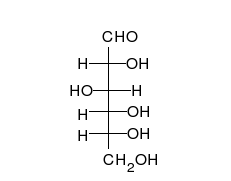# JEE Chemical Kinetics 2

Chemistry Level 2A reaction takes place in various steps. The rate constants for the first, second, third and fifth steps are $k_1,k_2,k_3$ and $k_5,$ respectively. The overall rate constant is given by $k=\dfrac{k_2}{k_3}\left (\dfrac{k_1}{k_5}\right )^{0.5}.$

If the activation energies are 40, 60, 50 and 10 kJ/mol, respectively, what is the overall energy of activation (in kJ/mol)?

Note: The above image is not the described reaction.

×